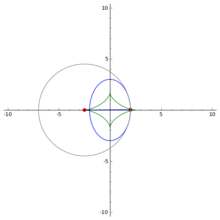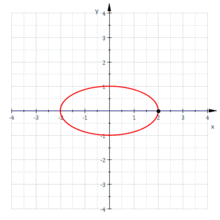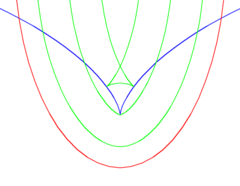# EvoluteAn ellipse (blue) and its evolute (green). The moving circle is the osculating circle to the ellipse, whose center is the center of curvature. It is also shown how the tangent line to the evolute is normal to the ellipse, i.e., the evolute is the envelope of the normals to the ellipse. The evolute of an ellipse is called an astroid.The evolute of a curve (in this case, an ellipse) is the envelope of its normals.

In the differential geometry of curves, the evolute of a curve is the locus of all its centers of curvature. That is to say that when the center of curvature of each point on a curve is drawn, the resultant shape will be the evolute of that curve. The evolute of a circle is therefore a single point at its center.

Equivalently, an evolute is the envelope of the normals to a curve.

The evolute of a curve, a surface, or more generally a submanifold, is the caustic of the normal map. Let M be a smooth, regular submanifold in Rn. For each point p in M and each vector v, based at p and normal to M, we associate the point p + v. This defines a Lagrangian map, called the normal map. The caustic of the normal map is the evolute of M.

## History

Apollonius (c. 200 BC) discussed evolutes in Book V of his Conics. However, Huygens is sometimes credited with being the first to study them (1673). Huygens formulated his theory of evolutes sometime around 1659 to help solve the problem of finding the tautochrone curve, which in turn helped him construct an isochronous pendulum. This was because the tautochrone curve is a cycloid, and the cycloid has the unique property that its evolute is also a cycloid. The theory of evolutes, in fact, allowed Huygens to achieve many results that would later be found using calculus. 

## Definition

Let γ(s) be a plane curve, parameterized by its arclength s. The unit tangent vector to the curve is, by virtue of the arclength parameterization,and the unit normal to the curve is the unit vector N(s) perpendicular to T(s) chosen so that the pair (T,N) is positively oriented.

The curvature k of γ is defined by means of the equationfor each s in the domain of γ. The radius of curvature is the reciprocal of curvature:The radius of curvature at γ(s) is, in magnitude, the radius of the circle which forms the best approximation of the curve to second order at the point: that is, it is the radius of the circle making second order contact with the curve, the osculating circle. The sign of the radius of curvature indicates the direction in which the osculating circle moves if it is parameterized in the same direction as the curve at the point of contact: it is positive if the circle moves in a counterclockwise sense, and negative otherwise.

The center of curvature is the center of the osculating circle. It lies on the normal line through γ(s) at a distance of R from γ(s), in the direction determined by the sign of k. In symbols, the center of curvature lies at the point:As s varies, the center of curvature defined by this equation traces out a plane curve, the evolute of γ.

### General parameterizations

If γ(t) is given a general parameterization other than the parameterization by arclength, say γ(t) = (x(t), y(t)), then the parametric equation of the evolute can be expressed in terms of the radius of curvature R = 1/k and the tangential angle φ, which is the angle the tangent to the curve makes with a fixed reference axis [the x-axis]. In terms of R and φ, the evolute has the parametric equationwhere the unit normal N = (−sinφ, cosφ) is obtained by rotating the unit tangent T = (cosφ, sinφ) through an angle of 90°.

The equation of the evolute may also be written entirely in terms of x, y and their derivatives. SinceandR and φ can be eliminated to obtain for a parametrically defined function:## Properties

Arclength

Suppose that the curve γ is parameterized with respect to its arclength s. Then the arclength along the evolute E from s1 to s2 is given byThus, if the curvature of γ is strictly monotonic, thenEquivalently, denoting the arclength parameter of the curve E by σ,This follows by differentiation of the formulaand employing the Frenet identity N′(s) = k(s)T(s):whence(1)

from which it follows that dσ/ds = |dR/ds|, as claimed.

Unit tangent vector

Another consequence of (1) is that the tangent vector to the evolute E at E(s) is normal to the curve γ at γ(s).

Curvature

The curvature of the evolute E is obtained by differentiating E twice with respect to its arclength parameter σ. Since dσ/ds = |dR/ds|, it follows from (1) thatwhere the sign is that of dR/ds. Differentiating a second time, and using the Frenet equation N′(s) = k(s)T(s) givesAs a consequence, the curvature of E iswhere R is the (signed) radius of curvature and the prime denotes the derivative with respect to s.

Relation with involute

With an appropriate starting point, the involute of the evolute of a curve is the curve itself.

Intrinsic equation

If φ can be expressed as a function of R, say φ = g(R), then the Whewell equation for the evolute is Φ = g(R) + π/2, where Φ is the tangential angle of the evolute and we take R as arclength along the evolute. From this we can derive the Cesàro equation as Κ = g′(R), where Κ is the curvature of the evolute.

### Relationship between a curve and its evoluteAn ellipse (red), its evolute (blue) and some parallel curves. Note how the parallel curves touch the evolute where they have cusps

By the above discussion, the derivative of (X, Y) vanishes when dR/ds = 0, so the evolute will have a cusp when the curve has a vertex, that is when the curvature has a local maximum or minimum. At a point of inflection of the original curve the radius of curvature becomes infinite and so (X, Y) will become infinite, often this will result in the evolute having an asymptote. Similarly, when the original curve has a cusp where the radius of curvature is 0 then the evolute will touch the original curve.

This can be seen in the figure to the right: the blue curve is the evolute of all the other curves. The cusp in the blue curve corresponds to a vertex in the other curves. The cusps in the green curve are on the evolute. Curves with the same evolute are parallel.

A curve with a similar definition is the radial of a given curve. For each point on the curve take the vector from the point to the center of curvature and translate it so that it begins at the origin. Then the locus of points at the end of such vectors is called the radial of the curve. The equation for the radial is obtained by removing the x and y terms from the equation of the evolute. This produces (X, Y) = (−R sinφ, R cosφ) or## References

1. "Circle Evolute - Wolfram Mathworld". Retrieved 5 December 2012.
2. Arnold, V. I.; Varchenko, A. N.; Gusein-Zade, S. M. (1985). The Classification of Critical Points, Caustics and Wave Fronts: Singularities of Differentiable Maps, Vol 1. Birkhäuser. ISBN 0-8176-3187-9.
3. Yoder, Joella G. Unrolling Time: Christiaan Huygens and the Mathematization of Nature. Cambridge University Press, 2004.